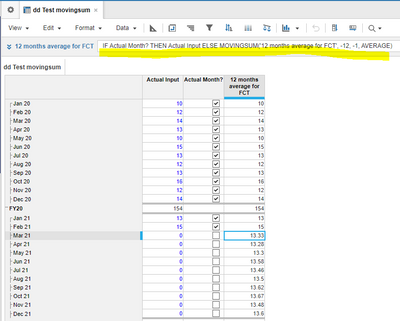# Movingsum formulaWhen using list versions, how do I make the movingsum formula include the forecast output for previous months in the calculation of a given months forecast??
I cant include a "previous" function within the movingsum formula

Tagged:

•This is the constraint that MOVINGSUM has. To achieve this functionality, break your formula in two different line items. In the first line item calculate the PREVIOUS value and in the second calculate the MOVINGSUM of the previous value.

~Anand

•@estebanmartinezanavate  the trick is to know that most of the Time functions, if you "look in the past", you can reference the line-item itself, including the MOVINGSUM function (like a recursive reference).

Below is an example to calculate the Forecast months as AVG of the previous 12 months:

in a line-item called '12 months average for FCT' you can put a formula like:

IF Actual Month? THEN Actual Input ELSE MOVINGSUM('12 months average for FCT', -12, -1, AVERAGE)

How to consider a month as Actual or not, there are different ways to do it...

You can have the same approach for this formula also when you use Versions.Hope it helps

Alex

•